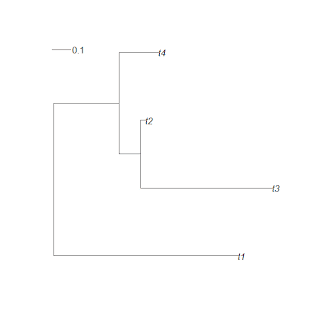## Monday, February 21, 2011

### Ancestral character estimation, with a trendIn response to the recent R-sig-phylo email listserve discussion thread on simulating Brownian evolution with a trend, Pasquale Raia inquired to the list about whether it would be straightforward to estimate ancestral states under this model. In practice, of course, it is generally not possible to fit the BM with a trend model (BMT for brevity in this post) - nor to estimate ancestral states under BMT - for conditions in which all the tips of the tree (and our data for trait values) are contemporaneous. However, for conditions in which the tips of the tree are effectively not contemporaneous (for instance, if we have fossil species in the tree or if the evolutionary process is punctuational), then we are in a theoretically much better position to estimate evolutionary trends.

The way I decided to approach this problem was very simple. First, let's consider the expected distribution of the data at tips and internal nodes. Well, we know from theory (and Gene Hunt's listserve post) that the theoretical distribution of tip and (by simple extension) internal node values under BMT is:

> x<-mvrnorm(n=1,mu=a+diag(C)*mu,Sigma=sig2*C)

Here, a is the root value, mu is the trend per unit time, and sig2 is the variance of the stochastic component of the model. C is a matrix of the heights above the root for each terminal and internal node in the tree (excluding the root). For example, in the 4 taxon phylogram plotted above, the matrix C is given by:

> C
t1     t3     t2     t4     6     7
t1 0.984819 0.0000000 0.0000000 0.0000000 0.0000000 0.0000000
t3 0.000000 1.1680555 0.4643410 0.3490977 0.3490977 0.4643410
t2 0.000000 0.4643410 0.4908593 0.3490977 0.3490977 0.4643410
t4 0.000000 0.3490977 0.3490977 0.5612080 0.3490977 0.3490977
6 0.000000 0.3490977 0.3490977 0.3490977 0.3490977 0.3490977
7 0.000000 0.4643410 0.4643410 0.3490977 0.3490977 0.4643410

Labels "t1", "t2", etc., correspond to tips of the tree; whereas "6", "7", etc., are internal node numbers from phy\$edge.

Now, for a given set of internal and terminal values, C, and values for a, mu, and sig2, we can calculate the probability density using dmnorm() in the {mnormt} package. Of course, that means we can also calculate the likelihood the ancestral states and evolutionary parameters, conditioned on the values for terminal species and the tree.

I have just put up (on my R-phylogenetics page, here) a very preliminary version of a function that uses the built-in R multivariate optimizer, optim(), to do this.

To test it out, I did the following.

First, I loaded fastBM() and anc.trend() from source:

> source("fastBM.R"); source("anc.trend.R")

Now, I simulated a random tree and data using rtree() from the {ape} package and fastBM():

> tree<-rtree(20)
> x.all<-fastBM(tree,a=0,mu=2,sig2=1,internal=TRUE)

Next, I estimated ancestral traits by maximizing the likelihood using anc.trend():

> result<-anc.trend(phy=tree,x=x.all[1:length(tree\$tip)], maxit=2000)

This function runs quite slow (not surprising, since in the above example I am doing a 22 dimension optimzation), so it has been somewhat hard to play around with so far. I can report that when optim() converges, mu is equivalent to the trend parameter estimated using fitContinuous(model="trend") in {geiger}.

In the above example, I obtained:

> result
\$ace
21     22     23     24     25     26
-0.2420098 0.1641928 1.2565491 3.0897363 1.8626764 1.0436854
27     28     29     30     31     32
2.0145277 3.7843751 4.8605234 6.3360333 1.7286019 2.3675538
33     34     35     36     37     38
3.1769088 5.3097681 3.3040749 2.1776491 0.2706769 0.9679458
39
1.1140074
\$mu
 1.693923
\$sig2
 0.3092271
\$logL
 -13.80569
\$convergence
 0
\$message
 "CONVERGENCE: REL_REDUCTION_OF_F <= FACTR*EPSMCH"

Although mu estimated in my function, and mu from fitContinuous() are the same; sig2 differed (invariably) by exactly n/(2n-2). This is the same as the ratio of the number of tips/(number of tips + number of nodes) in an unrooted, bifurcating tree.

In addition, the estimated ancestral states are fairly closely correlated with their known conditions. For instance, when I ran the code given above:

> cor(c(0,x.all[21:38):length(x.all)]),result\$ace)
 0.5973801

I should probably emphasize that the above results likely oversell the method because in general this cannot be used for species with only contemporaneous tips (rtree() tends to produce phylogenies with wildly non-contemporaneous tips); and furthermore the simulated trend in this example was quite strong. Nonetheless - this is cool!